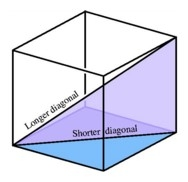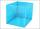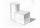# Cube

The sum of all cube edges is 30cm. Find the surface area of the cube.

S =  37.5 cm2

### Step-by-step explanation:Did you find an error or inaccuracy? Feel free to write us. Thank you!

Showing 1 comment:Dr Math
>>> Is the sum of all edges 30 or 80?

typo fixed, thank you!## Related math problems and questions:

• Cube 1-2-3Calculate the volume and surface area of the cube ABCDEFGH if: a) /AB/ = 4 cm b) perimeter of wall ABCD is 22 cm c) the sum of the lengths of all edges of the cube is 30 cm.
• Cube edgesThe sum of the lengths of the cube edges is 42 cm. Calculate the surface of the cube.
• Sum of the edgesThe sum of the lengths of all edges of the cube is 72 cm. How many cm2 of colored paper are we going to use for sticking?
• Cube 5The surface of the cube is 15.36 dm2. How will change the surface area of this cube if the length of the edges is reduced by 2 cm?
• Area to volumeIf the surface area of a cube is 486, find its volume.
• Cube volumeThe cube has a surface of 384 cm2. Calculate its volume.
• Cube edgeDetermine the edges of the cube when the surface is equal to 37.5 cm square.
• Cube wallsFind the volume and the surface area of the cube if the area of one of its walls is 40 cm2.
• Prism XThe prism with the edges of the lengths x cm, 2x cm, and 3x cm has volume 20250 cm3. What is the area of the surface of the prism?
• Cube 5The content area of one cube wall is 32 square centimeters. Determine the length of its edges, its surface and volume.
• Cube 7Calculate the volume of a cube, whose sum of the lengths of all edges is 276 cm.
• Wall diagonalCalculate the length of wall diagonal of the cube whose surface is 384 cm square.
• Cuboid - edgesThe cuboid has dimensions in ratio 4: 3: 5, the shortest edge is 12 cm long. Find: (A) the lengths of the remaining edges, (B) the surface of the cuboid, (C) the volume of the cuboid
• The blockThe block, the edges formed by three consecutive GP members, has a surface area of 112 cm2. The sum of the edges that pass through one vertex is 14 cm. Calculate the volume of this block.
• The cubeThe cube has a surface area of 486 m ^ 2. Calculate its volume.
• Surface area of cylinderDetermine the lateral surface of the rotary cylinder which is circumscribed cube with edge length 5 cm.
• Surface of cuboidFind the surface of the cuboid if its volume is 52.8 cm3 and the length of its two edges is 2 cm and 6 cm.